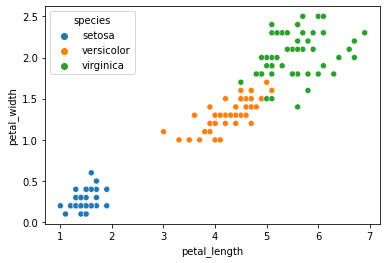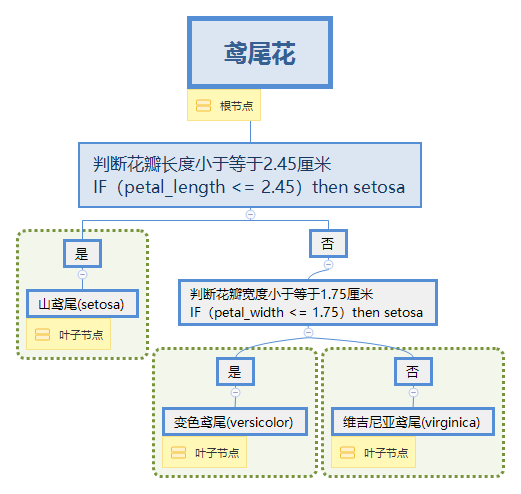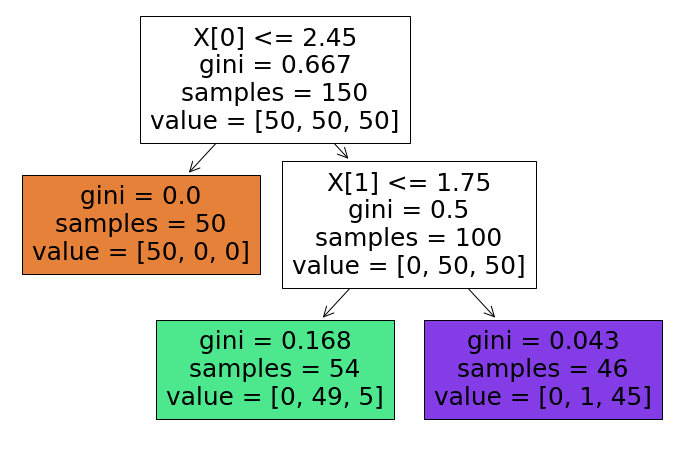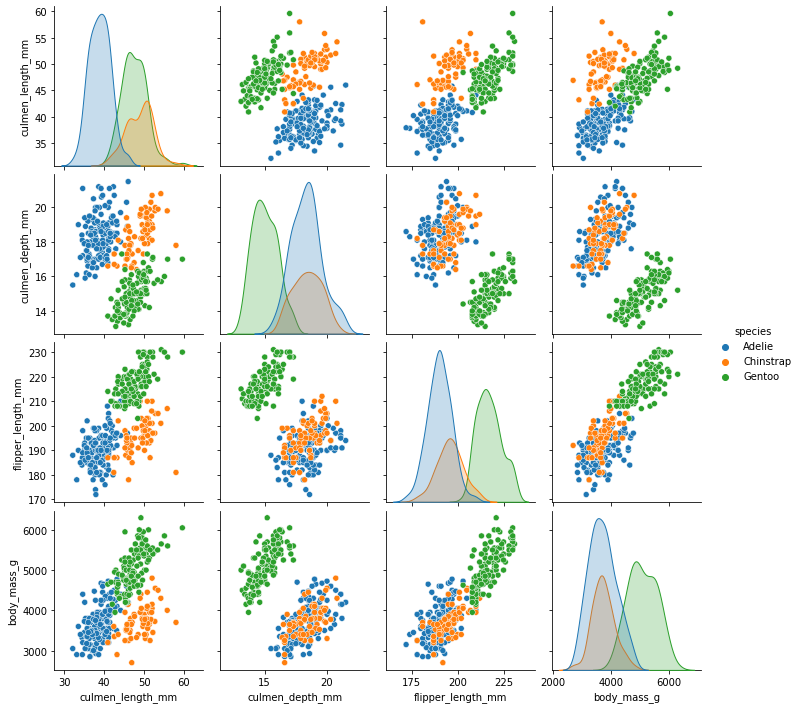# 一、决策树

## 1.1 什么是决策树## 1.2 决策树代码实践

import numpy as np
import pandas as pd
from sklearn.tree import DecisionTreeClassifier

#引入数据集

#决策树模型
X = df[['petal_length','petal_width']].to_numpy()
y = df['species']
tree_clf = DecisionTreeClassifier(max_depth=2, random_state=42)
tree_clf.fit(X, y)


import matplotlib.pyplot as plt
from sklearn.tree import plot_tree
plt.figure(figsize=(12,8))
plot_tree(tree_clf,filled=True);## 1.3 基尼不纯度

$G_i = 1 - \sum_{k=1}^{n}{p^2_{i,k}}$

# 二、随机森林

## 2.2 随机森林代码实践

### 2.2.1. 引入新的数据集

import numpy as np
import pandas as pd
import matplotlib.pyplot as plt
import seaborn as sns


df = pd.read_csv("https://blog.caiyongji.com/assets/penguins_size.csv")
df = df.dropna()

species island culmen_length_mm culmen_depth_mm flipper_length_mm body_mass_g sex
Adelie Torgersen 39.1 18.7 181 3750 MALE
Adelie Torgersen 39.5 17.4 186 3800 FEMALE
Adelie Torgersen 40.3 18 195 3250 FEMALE
Adelie Torgersen 36.7 19.3 193 3450 FEMALE
Adelie Torgersen 39.3 20.6 190 3650 MALE

• 特征：所在岛屿island、鸟喙长度culmen_length_mm、鸟喙深度culmen_depth_mm、脚蹼长度flipper_length_mm、体重(g)、性别

### 2.2.2 观察数据

sns.pairplot(df,hue='species')### 2.2.3 预处理

X = pd.get_dummies(df.drop('species',axis=1),drop_first=True)
y = df['species']


culmen_length_mm culmen_depth_mm flipper_length_mm body_mass_g island_Dream island_Torgersen sex_FEMALE sex_MALE
39.1 18.7 181 3750 0 1 0 1
39.5 17.4 186 3800 0 1 1 0
40.3 18 195 3250 0 1 1 0
36.7 19.3 193 3450 0 1 1 0
39.3 20.6 190 3650 0 1 0 1

### 2.2.4 训练数据

#训练
from sklearn.model_selection import train_test_split
from sklearn.ensemble import RandomForestClassifier
X_train, X_test, y_train, y_test = train_test_split(X, y, test_size=0.3, random_state=101)
model = RandomForestClassifier(n_estimators=10,max_features='auto',random_state=101)
model.fit(X_train,y_train)

#预测
from sklearn.metrics import accuracy_score
preds = model.predict(X_test)
accuracy_score(y_test,preds)


from sklearn.model_selection import GridSearchCV

param_grid = {'n_estimators':[10,15,20,25,30,35,40], 'learning_rate':[0.01,0.1,0.5,1], 'algorithm':['SAMME', 'SAMME.R']}
grid.fit(X_train,y_train)
print("grid.best_params_ = ",grid.best_params_,", grid.best_score_ =" ,grid.best_score_)


grid.best_params_ =  {'algorithm': 'SAMME', 'learning_rate': 1, 'n_estimators': 20} , grid.best_score_ = 0.9914893617021276